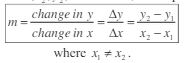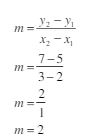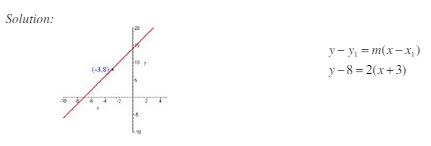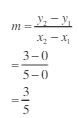Ontario Tech acknowledges the lands and people of the Mississaugas of Scugog Island First Nation.

We are thankful to be welcome on these lands in friendship. The lands we are situated on are covered by the Williams Treaties and are the traditional territory of the Mississaugas, a branch of the greater Anishinaabeg Nation, including Algonquin, Ojibway, Odawa and Pottawatomi. These lands remain home to many Indigenous nations and peoples.

We acknowledge this land out of respect for the Indigenous nations who have cared for Turtle Island, also called North America, from before the arrival of settler peoples until this day. Most importantly, we acknowledge that the history of these lands has been tainted by poor treatment and a lack of friendship with the First Nations who call them home.

This history is something we are all affected by because we are all treaty people in Canada. We all have a shared history to reflect on, and each of us is affected by this history in different ways. Our past defines our present, but if we move forward as friends and allies, then it does not have to define our future.

# Equations of Lines

## Calculating Slope

Any point on the xy-plane can be written as an ordered pair in the form (x, y). Taking two different points (x1,y1) and (x2, y2) on the line, the slope is:Example: Find the slope of the line through the points (2, 5) and (3,7).

Solution:## Equation of a line: Point-slope form

There are two common ways to represent a line. The point-slope form of the equation of a line is given by y – y1 = m(x – x1), where the line has a slope m and passes through the point (x1, y1).

Example: Write the equation of the line using point-slope form, given that the line passes through the point (-3, 8) and has a slope of 2. Also, graph the line.

Solution:## Equation of a line: Slope-intercept form

Slope-intercept form of the equation of a line is given by y = mx + b, where the line has a slope m and a y-intercept b. The y-intercept is simply the place where the line crosses the y-axis. The corresponding ordered pair denoting the y-intercept is (0, y1).

Example: Write the equation of the line with slope -3 and y-intercept 13 using the slope-intercept form.

Solution:

y = mx + b

y = -3x +13

Example: Find the equation of the line through the point (5, 3) and (0, 0).

Solution:

First, we find the slope:Next, we find the equation of the line:

y - y1 = m(x – x1)

y – 0 = 3/5 (x – 0)

y = 3/5x

Therefore, the equation of the line is y = 3/5x.

Equations of a Line: Given Two Points

Equations of a Line: Parallel Lines

Equation of a Line: Parallel Lines #2

Equations of a Line: Perpendicular Lines

Equation of a Line: Perpendicular Lines #2